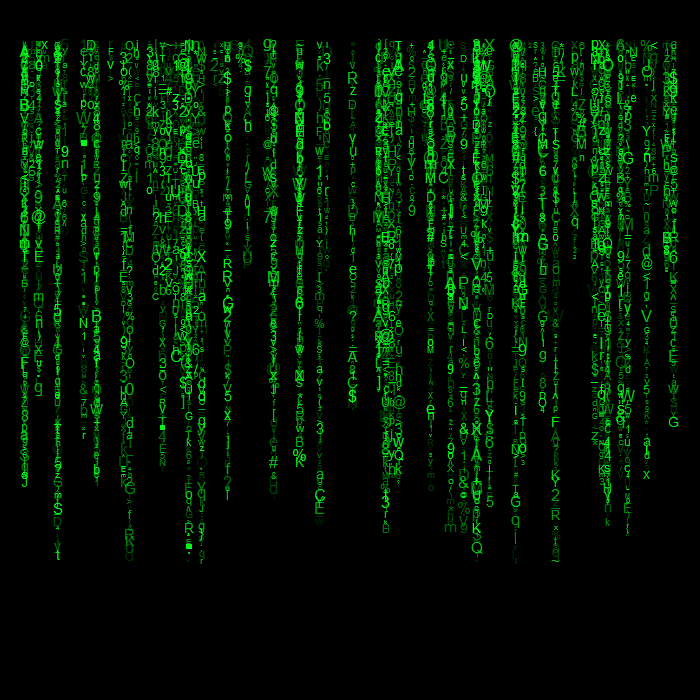Want to share your content on R-bloggers? click here if you have a blog, or here if you don't.

This post shares short code snippet to make your own screen saver in R, The Matrix-style:The code takes a few seconds to complete.

```nx = 100
ny = 80
kk <- 110
x = sample(x = 1:nx, size = kk, replace = TRUE)
y = seq(-1, -ny, length = kk)
codes <- matrix(0:127, 8, 16, byrow = TRUE, dimnames = list(0:7, c(0:9, letters[1:6])))
library(magrittr)
ascii <- c(apply(codes, c(1, 2), intToUtf8), letters, 1:10)
ascii <- sort(ascii)
ascii <- ascii[-c(1:37)]
# plot
op = par(bg = "black", mar = c(0, 0, 0, 0) )
plot(1:nx, seq(-1, -nx), type = "n", xlim = c(1, nx), ylim = c(-ny+10, 1), bty= "n", xaxt= "n", yaxt= "n")
for (i in seq_along(x) ) {
aux = sample(1:ny, 1)
points( rep(x[i], aux), y[1:aux],
pch = sample(ascii, aux, replace = TRUE),
col = hsv(0.35, 1, 1, runif(aux, 0.1)), cex = runif(aux, 0.3) )
}```

Because of randomness, once you ran the code, you will never see the exact screen saver elsewhere. Enjoy.